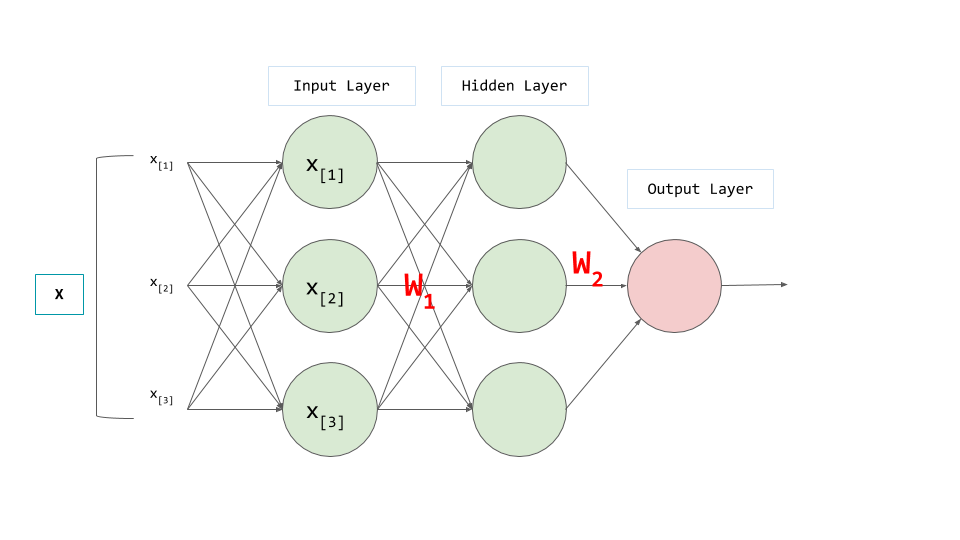# Activation functions in a deep neural networks

By Nischal Lal Shrestha | On Wed, Oct 14 2020 | Filed under Code

## Why do we need an Activation Function?

Let's have a look at a simple neural network.The output of the first layer will be

$$y_1 = W_1X_1 + b_1$$ $$y_2 = W_2(y_1) + b_2$$ $$y_2 = W_2W_1X_1 + W_2b_1 + b_2$$ $$y_2 = WX + b$$

which is linear output equivalent of using a single neuron. A single neuron is not powerful enough to do complex task. So, eventhough we are using a deep neural network with many layers it became equivalent of using a simple single neuron.

To prevent this collapse, we need beyond multiplication and addition. We will use what commonly known as activation function or transfer function. This activation function prevents collapsing because it adds a extra bit of processing before transfering the output of a neuron. Let's say we have an activation function that allows passing of value > 0. If any neuron have value < 0 then their output are not passed to next layer. By doing these stuffs, activation function introduce non-linearlity.

The result of using these functions is that we keep our network of many neurons, rather than having it turn into just one neuron, and that makes all the difference.